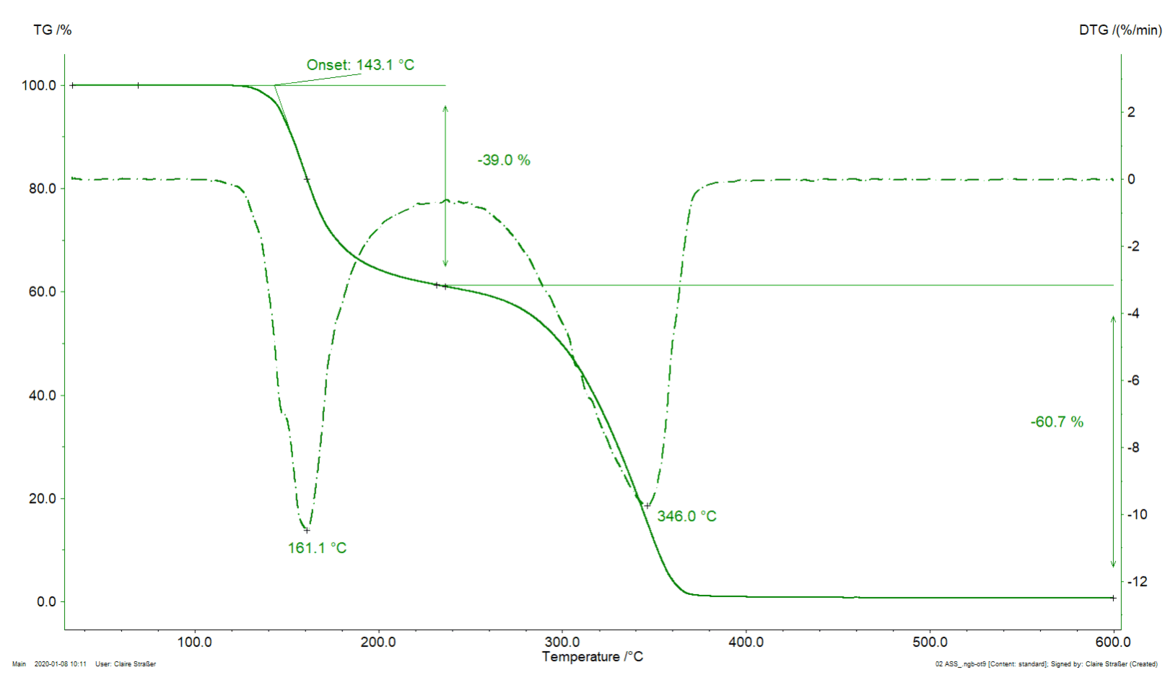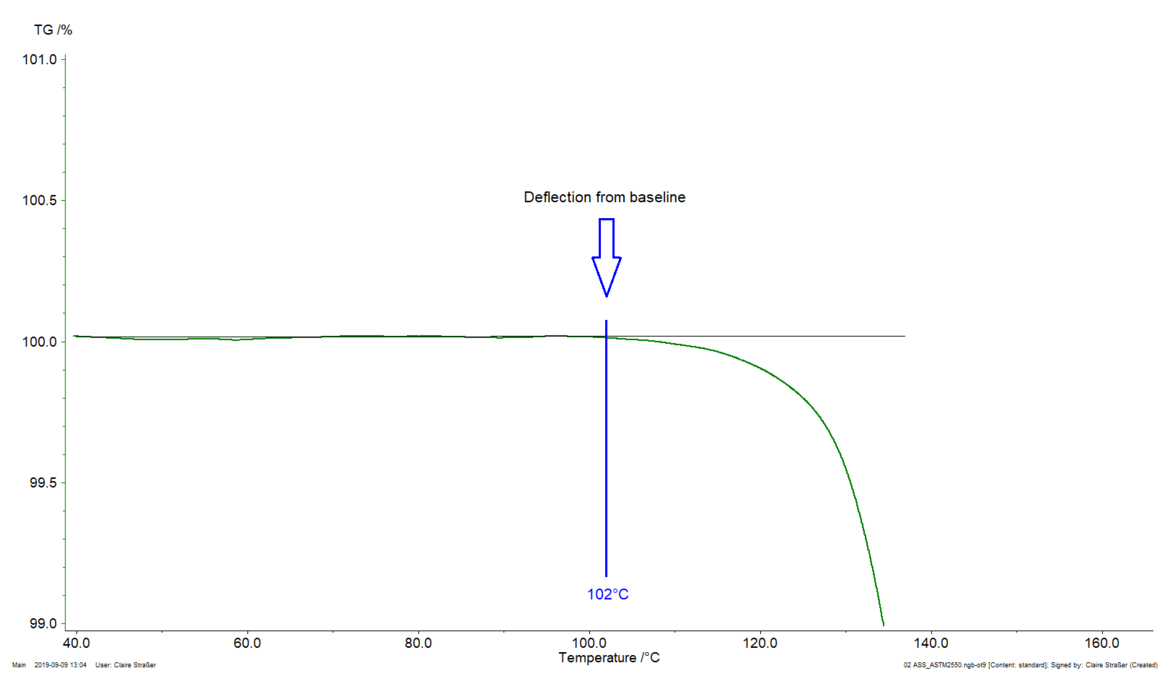Glossary

# Thermal Stability

A material is thermally stable if it does not decompose under the influence of temperature. One way to determine the thermal stability of a substance is to use a TGA (thermogravimetric analyzer).

The ASTM E2550 standard describes the thermal stability of a material as the “temperature at which the material starts to decompose or react, along with the extent of mass change determined using thermogravimetry”. It adds that “the absence of reaction or decomposition is used as an indicator for thermal stability”.

#### Example: Determination of the thermal stability of Aspirin®

Figure 1 displays the TGA curve of acetylsalicylic acid (better known as Aspirin®) during heating to 600°C in a nitrogen atmosphere. For more information about the decomposition mechanism of acetylsalicylic acid, please click here.

Two mass-loss steps were detected through the TGA curve (solid line). Each of these steps is evaluated through the determination of:

• Characteristic temperature at which the mass loss occurs
• Extent of mass change occurring during the step

Theoretically, three characteristic temperatures can be shown for a mass-loss step:

• Peak temperature of the DTG (1st derivative of the TGA curve, dash-dotted line)
• Extrapolated onset temperature according to the standard ISO 11358-1. This is “the point of intersection of the starting-mass baseline and the tangent to the TGA curve at the point of maximum gradient”
• Onset temperature according to ASTM E2550. This is the “point in the TGA curve where a deflection is first observed from the established baseline prior to the thermal event”

In the example presented, the first mass-loss step occurs at the following characteristic temperatures:

• 161°C (peak of the DTG curve, figure 1),
• 143°C (extrapolated onset temperature of the TGA curve, figure 1) or
• 102°C (onset temperature according to ASTM E2550, figure 2). This third value is used for evaluating the thermal stability of the tested acetylsalicylic acid sample.Figure 1. TGA measurement on acetylsalicylic acid, sample mass: 5.18 mg, crucible: aluminum oxide (open), heating to 600°C at 10 K/min in a dynamic nitrogen atmosphereFigure 2. TGA measurement on acetylsalicylic acid, zoom from figure 1. According to ASTM E2550, “the TGA curve should be zoomed to a scale of 1 mass% to 2 mass% for the onset temperature selection”.

Please note that the method is limited to materials that react or decompose in the investigated temperature range; it cannot be used for sublimation or VaporizationThe Vaporization of an element or compound is a phase transition from the liquid phase to vapor. There exists two types of vaporization: evaporation and boiling.vaporization.

#### Notes regarding the measurement conditions:

As the results are affected by the sample mass, the atmosphere (gas and flow rate), the heating rate and the crucible type, it is crucial to define the measurement conditions. For the same reason, the results for two samples can only be compared if the measurements were carried out under identical conditions.

The following measurement conditions are recommended:

1. Sample mass: between 1 and 10 mg, for example, 5 mg
2. Heating rate: 10 to 20 K/min (lower for energetic reactions: 1 to 10 K/min)
3. Flow rate of the atmosphere: 20 to 100 ml/min

In the example presented, the thermal stability is given at 102°C for a measurement on acetylsalicylic acid in a dynamic nitrogen atmosphere (gas flow: 40 ml/min), carried out on a 5-mg sample in an open aluminum oxide crucible and at a heating rate of 10 K/min.